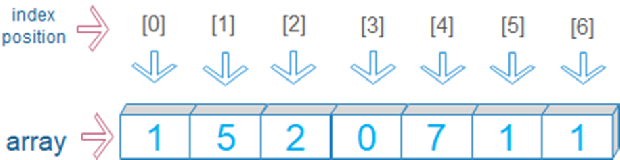# Creating arrays in PHPThree types of array
1. Indexed or Numeric Array
2. Associative Array
3. Multidimensional Array

## Indexed array

We will create an array and assign multiple values to this array like this
``\$my_data = array('mydata1','mydata2','mydata3', 'Mydata');``
In this array the index associated with each element is incremental numbers starting from 0.``echo \$my_data // mydata3``

## Associative Array

We can create an array with assigned Key ( indices ) and values like this. Note the use of `=>` operator to associate key with its value.
``````\$var=array(013=>"RKt",005=>"Bin",007=>"Alen");// Array key with value
"City"=>"Rain Town","Country"=>"USA");``````
Output from this array is here,
``````echo \$var; // Alen
echo "<br>";
echo \$ar["City"]; // Rain Town``````
Multidimensional arrays are discussed here.

## Creating array by breaking string

We can create an array by breaking string. Let us try with some simple examples.
``````///Storing the sample paragraph in a variable /////
\$string='plus2net provides easy tutorials for learning web programming';

\$new_array=explode(" ",\$string); // create an array by breaking string

// Let us display all elements of the array. ///
while (list (\$key, \$val) = each (\$new_array)) {
echo "\$key -> \$val <br>";
}
``````
Here we have used space as delimiter and created the array by breaking the string. The output of above code is here.
``````0 -> plus2net
1 -> provides
2 -> easy
3 -> tutorials
4 -> for
5 -> learning
6 -> web
7 -> programming``````
Now let us try another program. This is a keyword list, we will try to create array by breaking the string with coma as delimiter. Only changes to above code is shown here
``````\$string='scripts , languages, programs, forms, database';
\$new_array=explode(",",\$string);
``````
Output is here
``````0 -> scripts
1 -> languages
2 -> programs
3 -> forms
4 -> database ``````
If you want to know how many keywords are present by using array_size then here is the code.
``echo "Total Number of words = ".sizeof(\$new_array);``
Output of this is here
``Total Number of words = 5``

## Creating array by using range()

We can specify a range and step to create an array by using numbers or chars between the range.
``````\$my_array=range(0,10); // create an array
foreach (\$my_array as \$element) {
echo \$element . "  ";
}``````
Output
``0 1 2 3 4 5 6 7 8 9 10``

Subscribe to our YouTube Channel here

## Subscribe

* indicates required
Subscribe to plus2netplus2net.com